Subsections

# Many-Particle Systems

## General Problem

Suppose we have N identical particles 1, 2,.... We want to describe their thermodynamics--i. e. calculate partition function.

Mixtures:
N1 particles of kind 1, N2 particles of kind 2, ....

General formula:
Gibbs law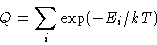(1)
What is a state in this formula?

I have a number of quantum states for each particle. The state is the set of occupation numbers n1, n2, ...
Consequence:
For quantum mechanics the grand canonical ensemble and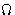potential are more natural than canonical ensemble and free energy!
I need coordinate qi and momentum pi for each of my particles plus information about the internal degrees of freedom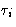for each particle.
We will discuss only classical case.

## Energy for a Classical System

• Kinetic energy. In the non-relativistic case it depends only on the momenta: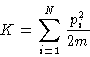• Potential energy. In the classical case it depends only on the coordinates: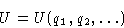• Internal energy. Depends on the internal degrees of freedom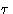(often they are quantum--even if everything else is classical!):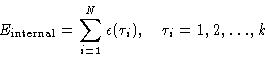## Same or Different Particles?

Boltzmann principle:
If the particles are identical, exchanging them will not change the state. This is a consequence of Quantum Mechanics.
For distinguishable particles classical partition function is: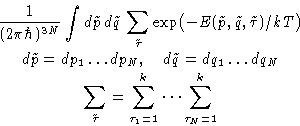However, they are identical. States are overcounted--divide by the number of permutations: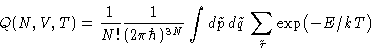(2)
This is called Boltzmann Statistics

## Factorization

Since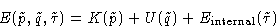we can write: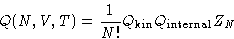(3)
Kinetic part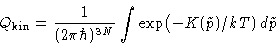Internal part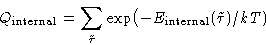Configuration integral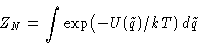## When This Does Not Work?

1.
Large quantum effects: we must include them honestly instead of as a kludge! But the kludge works for large temperatures (if the distance between quantum levels is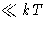)
2.
Relativistic effects: at velocities about light speed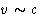or high gravitation fields we cannot separate kinetic and potential energy!
3.
Polarisability: sometimes we cannot separate U and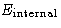.
4.
In non-Cartesian coordinates K might depend both on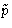and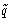--we cannot separate it! In the systems with rigid bonds we must use non-Cartesian coordinates.

## Kinetic part. Maxwell Distribution

Sincethe kinetic part is fully factorizable: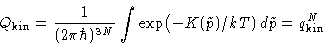with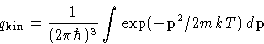Vector momentum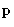: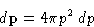and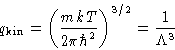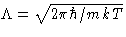has the dimension of length. It is called thermal de Brogile wavelength.

Quantum interpretation:
particles have wavelengths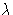depending on the momentum p. Thermal wavelength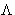corresponds to.
Validity of Boltzmann Distribution:
Classical formulae work if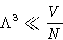Since partition function is factorized, the probability to have momentum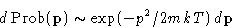For velocity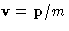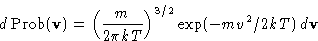or, since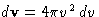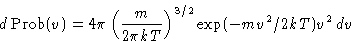This is called Maxwell distribution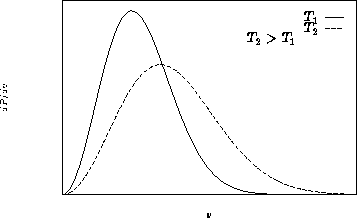Average velocity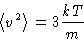Average kinetic energy per molecule: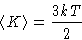Another definition of temperature: in classical systems measures the average kinetic energy of thermal motion.

In classical systems kinetic part can be separated from other parts and calculated exactly!

## Internal Part

The exact value depends on the molecular structure.

It is always factorizable: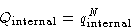with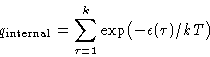anddepends only on T!

## Epitome

The kinetic part is calculated exactly--for all classical systems.

Calculation of the internal part is mostly a Quantum Mechanics problem.

Classical Statistical Thermodynamic is about calculation of the configuration integralNext: Ideal Gas Up: Systems with Many Particles. Previous: Systems with Many Particles.

© 1997 Boris Veytsman and Michael Kotelyanskii
Wed Sep 17 22:58:45 EDT 1997